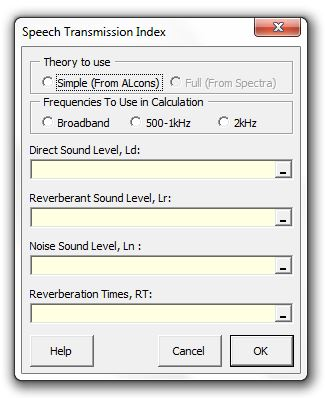### Strutt Help

Speech Transmission Index    1/1,1/3

Strutt|Electroacoustics|STI - Select the row of the direct sound level, reverberant sound level, noise sound level and reverberation time in the pop-up menu and Strutt will calculate the speech transmission index (STI) and insert the result into the active row of the worksheet.STI is calculated from the Percentage Articulation Loss of Consonants metric (%ALcons) via the Farrell-Becker Conversion:

STI = 0.9482 - 0.1845ln(%AL_(cons))

%ALcons is calculated using the following formula:

%AL_(cons) = 100 (10^(-2(A + BC - ABC)) + 0.015)

where,
A = -0.32 log_10((l_R + l_N) / (10l_D + l_R + l_N)) | A <= 1

B = -0.32 log_10(l_N / (10l_R + l_N)) | B <= 1

C = -0.5 log_10((RT)/12)

The relevant factors are the power ratios rather than the sound levels, where:

l_D = 10^(L_D//10) is the direct level power ratio

l_R = 10^(L_R//10) is the reverberant level power ratio

l_N = 10^(L_N//10) is the ambient level power ratio

RT is the reverberation time, s